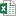## Itemprobabilities not updating

##### Itemprobabilities not updating
 Author Message Lizzyposted Last Year ANSWERGroup: Forum Members Posts: 23, Visits: 99 G'day,I'm programming an experiment that is like a lottery task, with two different lotteries, a red one and a blue one. You simply click on a lottery to play. The chances of 'winning' on each lottery need to change across the task, as well as the probability of getting a big versus small win.I thought about changing the probability of winning on each of the lotteries and the probabilities of getting a big versus small win by making a list for each lottery where a value is drawn, and based on that value trigger a win or loss, and through another list, trigger a big win or small win. Then I can set the itemprobabilities for those list items through values, and change those values at the start of each block.While the task runs however, the itemprobabilities are not changing. When I debug, I can see that the values that I plug into the itemprobabilities have changed in block2, but the data shows the probability of a win or loss being triggered actually hasn't changed. I've attached a simplified version of the script that has the same issue. Even though at the start of block2 I try to set the probability of a red win at .1 , and the probability of a blue win at .9, the data (monkey file also attached) shows that the chances of winning for red are .75, and for blue .25, which are the probabilities set at the start of the experiment. Similarly, the probabilities of a big versus small win are not being updated.Any idea where I might be going wrong?                                         AttachmentsTest datafile.xlsx (50 views, 124.00 KB)Item probabilities change test.iqx (54 views, 4.00 KB) Daveposted Last Year ANSWERGroup: Administrators Posts: 12K, Visits: 90K +x Lizzy - 4/26/2022G'day,I'm programming an experiment that is like a lottery task, with two different lotteries, a red one and a blue one. You simply click on a lottery to play. The chances of 'winning' on each lottery need to change across the task, as well as the probability of getting a big versus small win.I thought about changing the probability of winning on each of the lotteries and the probabilities of getting a big versus small win by making a list for each lottery where a value is drawn, and based on that value trigger a win or loss, and through another list, trigger a big win or small win. Then I can set the itemprobabilities for those list items through values, and change those values at the start of each block.While the task runs however, the itemprobabilities are not changing. When I debug, I can see that the values that I plug into the itemprobabilities have changed in block2, but the data shows the probability of a win or loss being triggered actually hasn't changed. I've attached a simplified version of the script that has the same issue. Even though at the start of block2 I try to set the probability of a red win at .1 , and the probability of a blue win at .9, the data (monkey file also attached) shows that the chances of winning for red are .75, and for blue .25, which are the probabilities set at the start of the experiment. Similarly, the probabilities of a big versus small win are not being updated.Any idea where I might be going wrong?                                        If you change the values responsible for the probabilities like this/ onblockbegin = [list.Acceptance_Red.resetselection(); list.Acceptance_Blue.resetselection()]/ onblockbegin = [values.acceptchance_red = .1; values.rejectchance_red = .9; values.acceptchance_blue = .9; values.rejectchance_blue = .1]/ onblockbegin = [values.winbig_red = 0.2; values.winsmall_red = 0.8]/ onblockbegin = [values.winbig_blue = 0.9; values.winsmall_blue = 0.1]/ trials = [1= StartTrial]/ timeout = 60000the lists won't pick that up unless you reset them after, i.e./ onblockbegin = [list.Acceptance_Red.resetselection(); list.Acceptance_Blue.resetselection()]/ onblockbegin = [values.acceptchance_red = .1; values.rejectchance_red = .9; values.acceptchance_blue = .9; values.rejectchance_blue = .1]/ onblockbegin = [values.winbig_red = 0.2; values.winsmall_red = 0.8]/ onblockbegin = [values.winbig_blue = 0.9; values.winsmall_blue = 0.1]/ ontrialbegin = [    list.Acceptance_Blue.reset();    list.Acceptance_Red.reset();    list.Winnings_Blue.reset();    list.Winnings_Red.reset();]/ trials = [1= StartTrial]/ timeout = 60000/ blocks = [1 = sequence(Block1, Block2)]If you do that, you should see a marked change in the probabilities between the two blocks. Example data file attached, the trial counts in that are:Block 1:AcceptedBlue = 30AcceptedRed = 80NotAcceptedBlue = 89NotAcceptedRed = 28Winnings_Blue = 30Winnings_Red = 80Red Acceptance = 80/(80+28) = 0.74Blue Acceptance = 30/(30+89) = 0.25Block 2:AcceptedBlue = 103AcceptedRed = 15NotAcceptedBlue = 8NotAcceptedRed = 97Winnings_Blue = 103Winnings_Red = 15Red Acceptance = 15/(15+97) = 0.13Blue Acceptance= 103/(103+8) = 0.93 AttachmentsItem probabilities change test_raw_1_2022-04-26-11-55-02-561.iqdat (51 views, 110.00 KB) Lizzyposted Last Year ANSWERGroup: Forum Members Posts: 23, Visits: 99 Amazing, that fixes the issue. Thank you so much! You're a legend Dave.

#### Merge Selected

Merge into selected topic...

Merge into merge target...

Merge into a specific topic ID...